# Solving functions for S in a q-q* Hamilton-Jacobi diffeq

## Homework Statement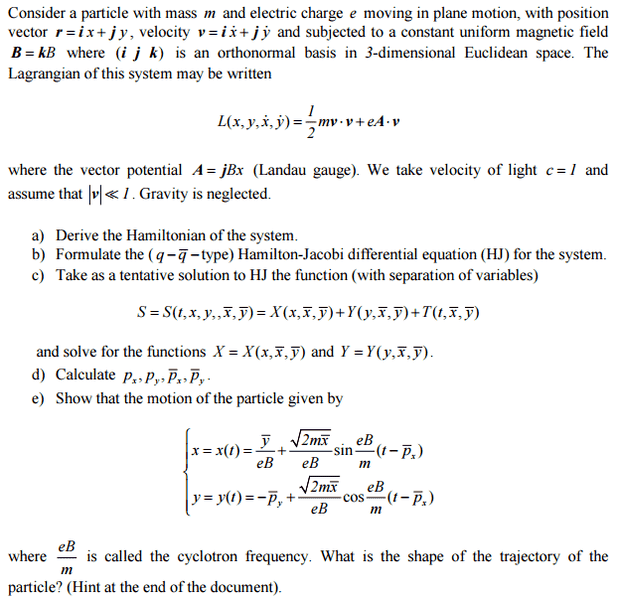## The Attempt at a Solution

So far I have a solution for a) as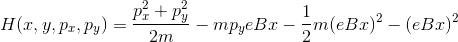For b) I formulate the equation as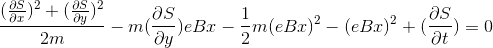and so far for c) I have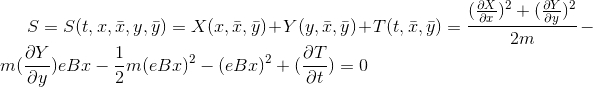My main idea at the moment is that as the Lagrangian was not time dependent, the Hamiltonian will not be. Following on maybe I can say that when separating variables T is constant so I can separate it so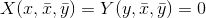but this is as far as I could get, as I couldn't think of how to separate them.

Any ideas, or are my problems due to a mistake in working?

TSny
Homework Helper
Gold Member

## The Attempt at a Solution

So far I have a solution for a) asThe last three terms do not have the correct dimesions. I believe you have dropped some factors of ##2## and ##m##. So, check your derivation.

Alternately, you might see if you can first take the Lagrangian as given in terms of a general vector potential ##\bf{A}## and show that the Hamiltonian can be written nicely as ##H = \frac{1}{2m} (\mathbf{p}-e \mathbf{A}) \cdot (\mathbf{p}-e \mathbf{A})##. Then substitute the given expression for ##\mathbf{A}##.
and so far for c) I haveYou probably didn't mean to have an equal sign after the ##T(t, \overline{x}, \overline{y})##

My main idea at the moment is that as the Lagrangian was not time dependent, the Hamiltonian will not be. Following on maybe I can say that when separating variables T is constant so I can separate it sobut this is as far as I could get, as I couldn't think of how to separate them.
You cannot conclude that ##T## is a constant. But you should be able to argue that ##\frac{\partial T}{\partial t}## is a constant.
Also, you should hope that ##X## and ##Y## are not identically equal to zero. If they were, there would be no hope of finding the correct equations for ##x(t)## and ##y(t)##.

Last edited:
My Hamilton was a mess from a mistake early on. After redoing it I get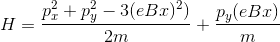.

I can then formulate the hamiltonian jacobi again and get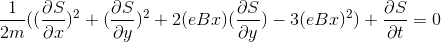Then (and I'm mostly going from an example in my lecture notes and the question hints) I can separate the variables out as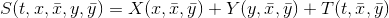That can be substituted as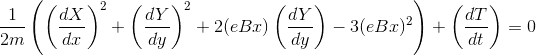In my notes the example only contains two separate variables e.g. X and T. He then makes every non time dependent term equal the time dependent terms and says they are both equal to a constant. He then solves X and T wrt this constant.

I'm not sure how to take this question further and solve for these functions.

TSny
Homework Helper
Gold Member
My Hamilton was a mess from a mistake early on. After redoing it I get.
The numerical factor of -3 is not correct for the ##(eBx)^2## term. Also, I believe the last term has the wrong sign.
Then (and I'm mostly going from an example in my lecture notes and the question hints) I can separate the variables out asYes
That can be substituted asIn my notes the example only contains two separate variables e.g. X and T. He then makes every non time dependent term equal the time dependent terms and says they are both equal to a constant. He then solves X and T wrt this constant.

I'm not sure how to take this question further and solve for these functions.

Here you want to use the usual type of reasoning when separating variables.
Suppose you have an equation of the form ##f(x) + g(y)## = 0 that must hold for all values of ##x## and ##y## within some domain. Here, ##f(x)## is a function only of ##x## and ##g(y)## is a function only of ##y##. Then you can argue that ##f(x)## must be a constant: ##f(x) = C## for all ##x##. Moreover, ##g(y)## must also be a constant which is the negative of ##C##; i.e., ##g(y) = -C##. Try to construct such an argument.

For your H-J equation, you have something of the form ##f(x,y) + \frac{dT(t)}{dt} = 0## that must hold for a range of values of ##x, y##, and ##t##. Use the type of argument mentioned above to deduce something about ##\frac{dT}{dt}##.

Okay, so I must have assumed something wrong early on or have made a pretty bad mistake in my work (not unheard of)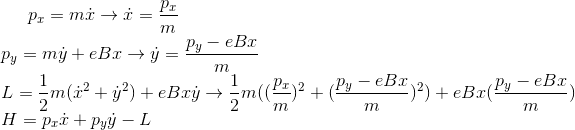TSny
Homework Helper
Gold Member
Okay, so I must have assumed something wrong early on or have made a pretty bad mistake in my work (not unheard of)So far so good. Just a matter of simplifying.

The Hamiltonian is the Legendre transformation of the Lagrangian:
## H = \sum_{i} \dot q_i \frac {\partial L} {\partial \dot q_i} - L = m(\dot x^2 + \dot y^2) + eBx\dot y - \frac {m} {2}(\dot x^2 + \dot y^2)- eBx\dot y##
## = \frac {1} {2m} (p_x^2 + p_y^2)##
In this problem you need to find constants of motion. If you solve for the Lagrangian eqns. of motion, you will find:
## m\ddot x -eB\dot y = 0 →\frac {d} {dt}(m\dot x -eBy) = 0## and
## m\ddot y +eB\dot x = 0→\frac {d} {dt}(m\dot y +eBx) = 0##
Thus we have the constants of motion:
##\tilde p_x =(p_x -eBy) ##,
##\tilde p_y =(p_y +eBx)##.
We write the HJ equation with ## T(t,\tilde x,\tilde y) = \tilde xt## and substitute ## p_y = \tilde p_y - eBx## to get:
## (\frac {dX} {dx})^2 + ( \tilde p_y - eBx)^2 = 2m \tilde x##,
## X= \int dx\sqrt { 2m \tilde x- ( \tilde p_y - eBx)^2}##
## S= \int dx\sqrt { 2m \tilde x- ( \tilde p_y - eBx)^2} - \tilde xt##
##p_x = \frac {\partial S} {\partial x} = \sqrt { 2m \tilde x- ( \tilde p_y - eBx)^2} ##
##\tilde p_x = -\frac {\partial S} {\partial \tilde x} = -m \int dx\frac {1} { \sqrt { 2m \tilde x- ( \tilde p_y - eBx)^2}} +t##
We now make the substitution in the integral:
## x' =\tilde p_y - eBx ##,
## dx' = -eBdx ##
##\tilde p_x = -\frac {\partial S} {\partial \tilde x} = \frac {m} {eB} \int \frac {dx'} { \sqrt { 2m \tilde x- x'^2}} +t = \frac {m} {eB}sin^{-1}(\frac{\tilde p_y - eBx} { 2m \tilde x}) + t##
You can do the algebra to solve for x in terms of ##\tilde p_x ,\tilde p_y## and ##\tilde x##.
To get y just use the constant of motion equation ## y = \frac {m\dot x - \tilde p_x} {eB}##